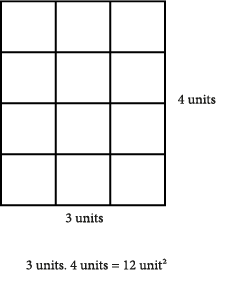## Posts

Showing posts from January, 2019

### IdentitiesIdentities Identities are ways of expressing algebraic values in different ways. The values of the written expressions are identical. This is why the word ‘identical’ is used. "Same thing Different form!" Such following expressions and equations are identical: $$\displaystyle 3x=2x+x$$ $$\displaystyle 5.(x+1)=5x+5$$ $$\displaystyle (a+b)²=a²+2ab+b²$$ Identities are valid / true for all real numbers.  Let us choose a number for this expression  $$\displaystyle 5.(x+1)=5x+5$$   and try.  Let  $$\displaystyle x=8$$  $$\displaystyle 5.(8+1)=5.8+5$$  $$\displaystyle 5.(9)=40+5$$ $$\displaystyle 45=45$$ The left side is 45, the right side is 45, and they are equal to one another. Whatever number you give $$\displaystyle x$$  the right and left side will equal to one another because the expressions are identical.  They are the same. Why do we need identities?  They can help us wh

### Modeling in IdentitiesModeling in Identities Calculation of area by means of multiplication is an effective visual representation.  Multiplication and Area  $$\displaystyle 3.4$$   ; If we multiply 3 with 4, we will have found the area (the number of squares) within a  rectangle, which has a length of 3 units.